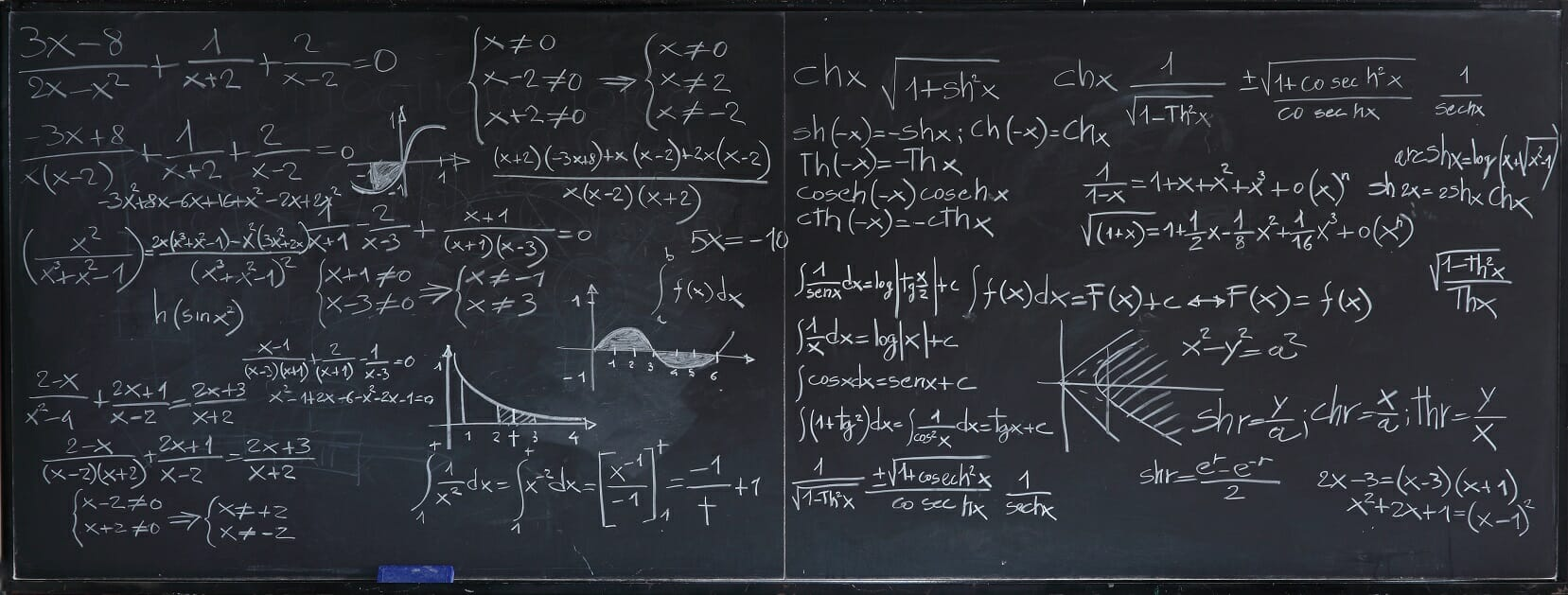# Financial Math Glossary

Important mathematical terms to know

## Financial Math Glossary

This financial math glossary covers the most important terms and definitions required for a career as a financial analyst.  This list is taken from CFI’s Financial Mathematics Course.###### Annuity

An annuity is a series of payments in equal time periods, guaranteed for a fixed number of years.

###### Annuity factor

The present value of \$1 paid for each of t periods.

###### Arithmetic mean

An average calculated by adding the value of the points in a data set and dividing the sum by the number of data points.

###### Constant Perpetuity

A constant stream of identical cash flows without end.

###### Correlation

A statistical measure of how two securities move in relation to each other.

###### Coupon Rate

Coupon Rate is the amount of interest received by a bond investor expressed on a nominal annual basis.

###### Covariance

A statistical measure of the variance of two random variables that are observed or measured in the same mean time period.

###### Current Yield

The coupon from a bond divided by the market price of the bond, expressed as a percentage.

###### Discount Factor

The percentage rate required to calculate the present value of a future cash flow.

###### Growing Perpetuity

A constant stream of cash flows without end that is expected to rise indefinitely.

###### Moving Average

The average of time-series data from several consecutive periods. It is called a ‘moving’ average because the average is constantly recalculated once new data becomes available for the next period.

###### Par Value

Par Value is the amount returned to the bond investor by the issuer upon maturity.

###### R-Squared

R-Squared is a statistical term saying how good one term is at predicting another. Generally, a higher value of R-Squared means that you can better predict one term from another.

###### Regression Analysis

A statistics-based method that can be used in Excel that analyzes the relationship and the strength of correlation between a dependent variable and one or more independent variables.

###### Standard Deviation

A measure of how far a set of data is from the average. The further it is, the higher its standard deviation is. Standard deviation is computed by taking the square root of variance.

###### Sum of Squares

The Sum of Squares Regression (SSR) measures how much variation there is in the modeled values and this is compared to the Total Sum of Squares (SST), which measures how much variation there is in observed data, and to the Sum of Squares Residual (SSE), which measures the variation in the modeling errors.

###### Time Value of Money

The idea that money is worth more the sooner it is obtained, or if it is in the present. This is because money in the present can be used and invested to generate a return while money to be expected in the future cannot be used. Time value of money is affected by both the periods of time being considered as well as the discount rate for calculating present value.

###### Variance

Variance is a measure of the dispersion of a set of data points around their mean value. It is a mathematical expectation of the average squared deviations from the mean.

###### Weighted Average

An average in which some values count for more than others.

###### Yield to Maturity

The annual return earned by a bond investor if purchasing a bond today and holding it until maturity.

### More financial mathematics resources

This financial math glossary covered important terms and definitions for performing financial analysis.  Now you’re ready to take CFI’s Financial Mathematics Course. To continue learning and advancing your career, these resources will also be helpful:

• Fixed Income Terms
• Financial Ratios Terms
• Behavioral Finance Terms
• M&A Terms

### Financial Analyst Training

Get world-class financial training with CFI’s online certified financial analyst training program!

Gain the confidence you need to move up the ladder in a high powered corporate finance career path.

Learn financial modeling and valuation in Excel the easy way, with step-by-step training.